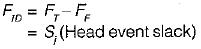Courses

# CPM & PERT - 2

## 10 Questions MCQ Test Mock Test Series for Civil Engineering (CE) GATE 2020 | CPM & PERT - 2

Description
This mock test of CPM & PERT - 2 for Civil Engineering (CE) helps you for every Civil Engineering (CE) entrance exam. This contains 10 Multiple Choice Questions for Civil Engineering (CE) CPM & PERT - 2 (mcq) to study with solutions a complete question bank. The solved questions answers in this CPM & PERT - 2 quiz give you a good mix of easy questions and tough questions. Civil Engineering (CE) students definitely take this CPM & PERT - 2 exercise for a better result in the exam. You can find other CPM & PERT - 2 extra questions, long questions & short questions for Civil Engineering (CE) on EduRev as well by searching above.
QUESTION: 1

Solution:
QUESTION: 2

Solution:
QUESTION: 3

### Match List-I with List-II and select the correct answer using the codes given below the lists: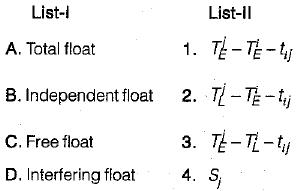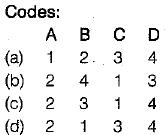Solution:
QUESTION: 4

From the network shown in the figure below (the number on each arrow denotes the time duration of activity in days), the earliest start time, in days for activity 5-6 is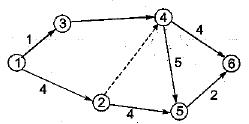Solution:
QUESTION: 5

In the network shown in figure total float for the activity 2-4 will be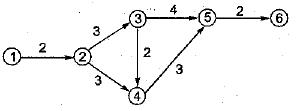Solution: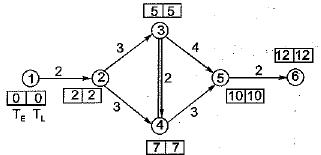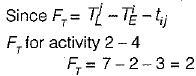QUESTION: 6

Probability of finishing of a project earlier than expected time is

Solution:

Probability of finishing a project within expected time is 50%.
Probability of finishing the project earlier than expected time probability is less th an 50%.

QUESTION: 7

The probability distribution taken to represent the completion time in PERT analysis is

Solution:

PERT analysis follows beta distribution.

QUESTION: 8

Whenever an activity has zero total float, then

Solution:
QUESTION: 9

A critical path has

Solution:

Minimum slack gives more critical path.

QUESTION: 10

Interfering float is the difference between

Solution: